# Split Date & Time into Separate Cells – Excel & Google Sheets

This tutorial will demonstrate multiple ways to split dates and times into separate cells.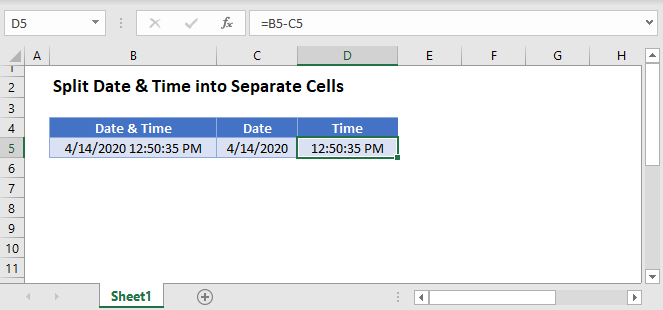## Dates and Times in Excel

In Excel, dates are stored as serial numbers where each whole number represents a unique date. Times are stored as decimal values. Look at this example: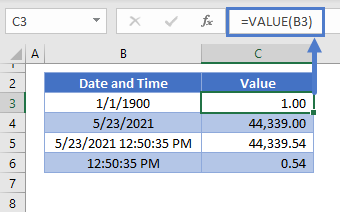Knowing how dates and times are stored in Excel, makes it easy to split them into separate cells.

## Split Dates and Times

To split dates and times, there are several functions that we can use to return the integer value of the date and time.

### INT Function

First we will use the INT Function to return the integer value representing the date:

``=INT(B3)``

###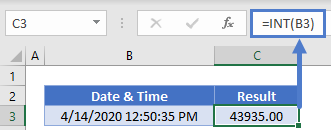TRUNC Function

Or we can use the TRUNC Function to trim off the decimal (time) value:

``=TRUNC(B3)``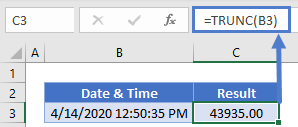### ROUNDDOWN Function

Or you can use the ROUNDDOWN Function to round down to the nearest whole integer (date):

``=ROUNDDOWN(B3,0)``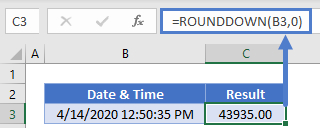### Time Value

Next we will subtract the integer (calculated using one of the above 3 methods) from the original date and time. The remainder will be the time:

``=B3-INT(B3)``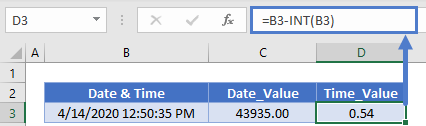### Easy Excel AutomationLast, go to the Home Ribbon > Number and change the cell formatting from General to Short Date (for the date) and Time (for the time):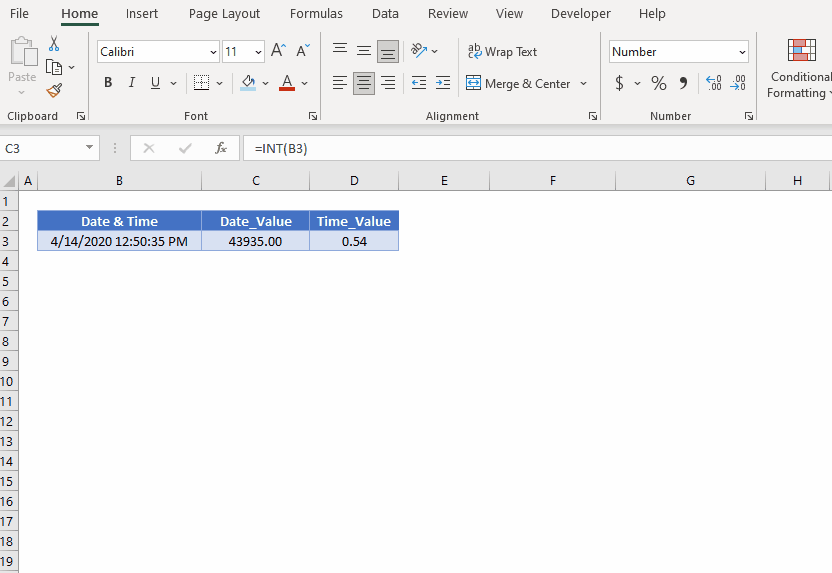Final Result Showing as below.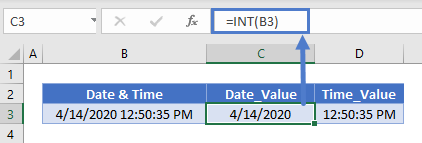## Split Date & Time With Formatting

You can also split the date and time into two separate cells by referencing the original cells and adjusting the formatting:

``=B3``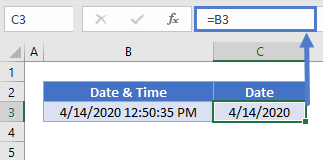``=B3``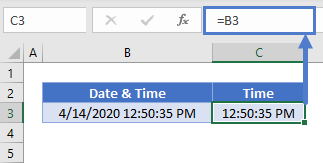However, when you adjust the formatting to show only the date and/or time, the original entire date & time remains in the cell as a serial number with a decimal.  The only difference is the final display to the user.

You can change the date format from the Cell Formatting Menu: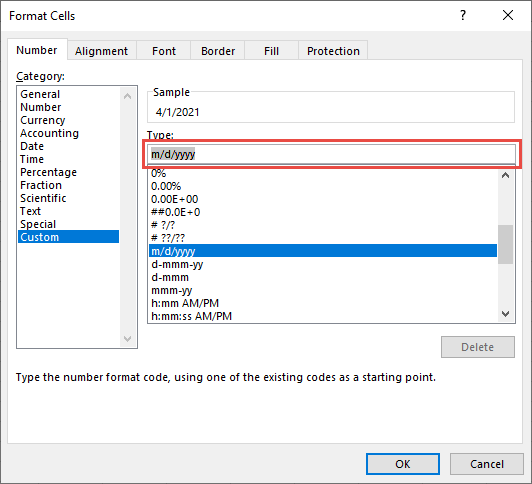Type “m/dd/yyyy” in the Type area to set date formatting, or “h:mm AM/PM” to set time formatting..

To access the Cell Formatting Menu use shortcut CTRL + 1 or press this button: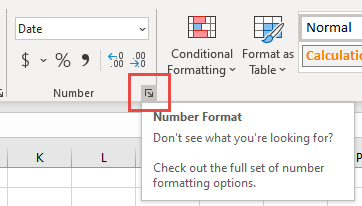### TEXT Function

You can also use the TEXT Function in separate cells to store the date and time values separately as text:

``=TEXT(B3,"m/d/yyyy")``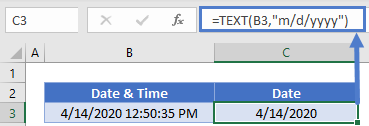``=TEXT(B3,"hh:mm:ss AM/PM")``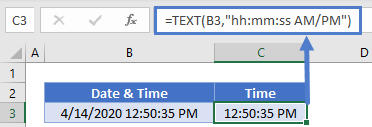However, these dates and times are now stored as text and the usual date and time math will no longer apply.

## Split Date & Time into Separate Cells in Google Sheets

All of the above examples work exactly the same in Google Sheets as in Excel.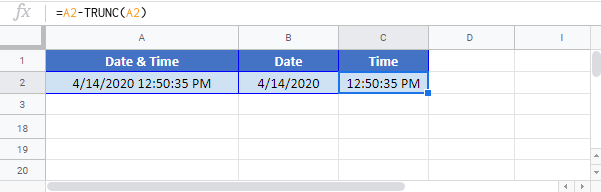### Excel Practice Worksheet

Practice Excel functions and formulas with our 100% free practice worksheets!